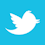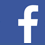In PCB transmission lines, a correct design can render leakage negligible.

There is a struggle going on related to building fast logic circuit boards. Designs fail because they radiate or will not function at the required clock rate. Explaining why one approach works and another fails brings out some strange explanations. Herein, we discuss this design problem using well-known principles. New science is not needed. New language is not needed. What will be said will probably surprise readers, however, as this area of electrical behavior is often poorly understood.

This article discusses the layout of circuit boards with clock rates that exceed 50MHz. We start by accepting a few basic ideas that come from field theory. These ideas are fundamental to all electrical behavior. These same ideas are the basis for the circuit theory readers are familiar with. These ideas are simple, and they work. They describe how nature stores and moves electrical energy. The voltages associated with this energy flow are the logic levels of interest. The biggest problem we face is that we cannot see or measure energy flow directly. Yes, we observe voltages, but that is only part of a bigger picture. In logic, a voltage can indicate static or moving energy. In some cases, static and moving energy occur together. A voltage does not indicate energy flow direction. Transmission lines permit reflections, and that is certainly not circuit theory. A lot happens during a rise or fall time. In fact, this is the only time there can be radiation. A wave front does not necessarily mean energy is in motion. In many cases it means energy is being stored or taken out of storage. In a first reflection, a wave travels in a direction opposite to energy flow direction. Understanding these details is key to understanding how logic is moved on a circuit board. There is a lot we cannot sense by measuring voltage. This lack leads to a lot of confusion.

##### The Fundamental Objective of Nature

Nature has one main objective: to reduce potential energy. This is why a rock falls, sound propagates or a chemical reaction takes place. The energy that arrives from the sun is mainly electromagnetic. The sun’s potential energy is reduced by evaporating ocean water, creating weather, growing crops and maintaining life. By doing work, the sun’s energy takes on new forms that have less potential to do work. We have learned how to direct this flow of energy to make our lives simpler. We store energy and then let it do work for us at a later time. For example, water in a dam turns a turbine that generates electricity that powers our cities. On a PCB we store energy in capacitors and use it to perform logic operations.

What we must understand is that nature is only interested in moving energy to a lower potential. If we use nature’s intent, we can let her perform logic functions that are free from error. When a logic switch closes, we are letting nature move some energy to a lower state. We must provide a path that is simple and direct. We measure this activity in terms of voltage. If the energy continues to rattle around in the circuit, then logic errors can result. Our interests are stable voltage levels at points in time and space. This is good engineering, and in turn, good logic.

To see how simple this energy flow idea is, consider a transmission line used to carry a logic signal. A logic level of 5V requires the capacitance of the line store energy equal to 1/2CV2. To return the logic voltage to zero, this energy must be dissipated. Thus, a logic signal is the presence or absence of energy stored on a transmission line. Performing logic operations on a PCB is the job of moving energy on and off of sections of transmission line. For slow logic rates, an energy viewpoint is unnecessary. Today, logic speeds require a change in approach.

We think of logic in terms of voltage. To see how energy moves, it is necessary to look at reflections and wave activity. Voltage differences indicate energy is present, but the direction of flow or whether the energy is static may not be obvious. Obtaining new energy for transport is also part of the problem. A fast logic board works when energy storage and flow is under control. Energy must be available at points of demand. Logic levels must be transported in spaces that carry little or no interference. Logic operations must not create interference. This means controlling cross-coupling is important. Reflections are needed and must be carefully used.

A lesson to be learned here is nature does not care what the energy represents. Besides logic, energy flow can operate a component, or it can be interference. One way to express this is “nature does not read labels.” Nature sees every conductor pair is a possible transmission line for anything that happens. This one fact is the basis for understanding many interference problems. We must provide conductor geometries that separate these functions. Correctly stated, clean logic requires the use of dedicated designed spaces. This avoids all cross-coupling.

##### Current Flow

The charge stored on the capacitance of a transmission line means there must have been current flow at some earlier point in time. This current flow implies a magnetic field, which is needed to move energy. The movement of energy into a capacitance thus requires both an electric and a magnetic field. These fields move energy in the space between conductors. What is critical in fast logic is the shape of the space carrying the energy must be controlled. Typically for logic, this space is a cylinder. If the energy space becomes a pancake (spreads out) then the energy is not contained, and there will be reflections, delays and possible board-edge radiation. This means via positions and via spacings are critical.

A simple transmission line is a pair of conductors. It can be a single trace over a conducting plane or a trace between two conducting planes. The energy carried in the initial wave is controlled by the ratio of inductance to capacitance per unit length. At a change in geometry, wave reflections can change the energy storage patterns. When there are uncontrolled reflections, there are delays. Ideally the voltage that arrives at a logic gate is at the correct value and remains a constant until the next logic level is received. The objective in board layout is to find ways to achieve this goal.

Transmission lines function down to DC. The practical way to have a controlled magnetic field at DC is to have current flow in conductors. In a transmission line carrying energy, the space carrying the energy must be bounded by a forward and return current. This is the only way there can be a magnetic field for logic. In coax, the return current path is a conducting sheath. On a logic circuit board, the return current often uses a conducting plane. In PCB transmission lines, the spacing between conductors is small, and most of the energy is contained under a trace. Yes, there is some field leakage, but if the design is correct, this leakage is small enough that it can be neglected. Depending on line length, the line must be terminated in its characteristic impedance. The simplest approach to termination is a series resistor at the logic source.

##### The Energy Dilemma

Schools of thought say the energy is carried by charges moving on conductors. To put this idea to rest, consider a 50Ω line and 5V logic. The current level is 0.1A. The number of electrons free to move on a typical trace is Avagadros’s number, or about 6 x 1023. At this current level, 1014 electrons move at about 3cm/sec. Only one electron in a trillion is moving. To put this into perspective, there are only 7.4 billion people on earth. One in a trillion means that essentially no electrons are moving. This leaves us with the question: What do we mean by current flow?

The movement of energy does not involve the movement of mass, charge or field. Here is an interesting analogy. In an automobile, the drive shaft carries energy in stress (torque) and rpm. It is interesting to note the velocity of energy in the shaft. Stress in steel travels at about 10,000mph. The torque and rpm do not move forward. In fact, these parameters are both vectors that are perpendicular to the shaft axis. The parallel with moving electrical energy is obvious. In a logic transmission line, the E and H vectors in classical physics are static and perpendicular to the direction of energy flow. In logic, these fields, even if unchanging, are coupled together and carry energy at the speed of light. For some reason this is very difficult to accept. Fields do not move when energy flows. Fields that carry energy are put into position by wave action.

##### Fast Circuit Boards – Energy Management

This is the title of my latest book published by John Wiley. It describes in detail how fields carry energy on logic circuit boards. The first chapter discusses the electric and magnetic fields that move energy. The second and third chapters discuss the transmission lines found on PCBs. This material includes vias, energy sources, decoupling capacitors and various conductor configurations. The transitions that take place at leading edges are explained in detail. There is some mathematics, but an attempt is made to explain everything using words. There are chapters on interference and radiation. A group of appendices cover decibels, abbreviations, circuit theory basics and the Smith Chart.

The methods described in this book have been put into practice, and they work. These methods suggest the directions that must be taken in the packaging of IC components. Moving logic is a systems problem that must be solved on many fronts. There is a lot of room for faster logic, and it requires understanding basic principles.

Ralph Morrison has 50 years of experience in electronics engineering and is author of eight books, including Solving Interference Problems in Electronics, Grounding and Shielding Techniques in Instrumentation, and The Fields of Electronics: Understanding Electronics Using Basic Physics; This email address is being protected from spambots. You need JavaScript enabled to view it..